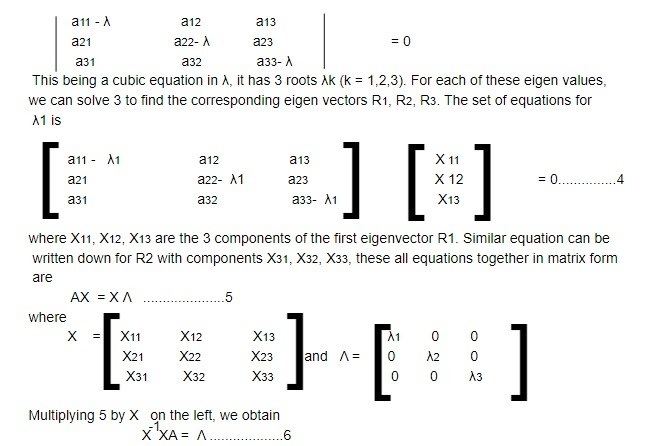# Euler's theorem

A rigid body is capable of two independent motions: rotation and translation. The position and orientation of such a body in motion can therefore be fully described. Three of these six degrees of freedom correspond to the body's translational motion, while the remaining three characterize its rotating motion. The general displacement of a rigid body with one fixed point is a rotation about some axis, according to Euler's theorem.

The body cannot move in translation because one point is fixed and is considered to be the origin of the body set of axes. Therefore, by using a single rotation of the initial set of axes, which may coincide with the space set of axes at the first time, we can always acquire the body set of axes. This is because the initial set of axes may coincide with the space set of axes at the initial time. Since we are aware that a vector's direction might change during a rotation but not its magnitude, Consequently, the challenge is to identify the rotational axis.

Let the rotation be brought about by a matrix A and if such a vector along the axis of rotation be R, then the transformed vector R' must be identical with R.

R' = AR = R....................1

This equation is of the form AR= λR, (λ = 1).......................................2

called an eigenvalue equation, where λ is a constant. If we are able to solve the equation for the matrix A, i.e. its eigenvalues λ, and the corresponding eigenvectors R are found, then the Euler's theorem is proved.

Equation 2 can also be written as

(A - λI)R = 0.........................3

where I is the identity matrix. For the solution to exist,

l A -λI l R = 0Λ is a diagonal matrix whose diagonal elements are the eigen values λ1, λ2, λ3 of the matrix A.

Equation 6 then represents the diagonals of the matrix through similarity transformation.

The matrix X that diagonalised A has along its columns the eigenvectors of A and the elements of the diagonalised form of A are the corresponding eigenvalues.

Now in order to represent a rigid rotation, the matrix A that performs this rotation has the following properties:

1. It must be an orthogonal matrix since an orthogonal matrix leaves the magnitude of any vector unchanged.This note is taken from Statistical mechanics, Msc Physics, Nepal.

This note is a part of the Physics Repository.

Previous topic : Rigid bodies- Kinematics and Dynamics

Next topic : Euler's angles